英雄的故事将要谢幕，似乎每段传奇都该有个华丽的结局。于是我打算用全新的魔法、炫酷的特效和再一次的重构为这期教程画上句号；虽然依旧伴随着一些客观因素导致的瑕疵，然而更让我欣慰的是这款快被敲烂了的Silverlight MMORPG客户端引擎正逐步迈向成熟。或许Silverlight 5 的新特性能给这款引擎带来质的飞跃，又或者我们更加期待Web 3D 游戏时代的来临，那望眼欲穿的公元2011年春

14.1完结篇[一期] (交叉参考：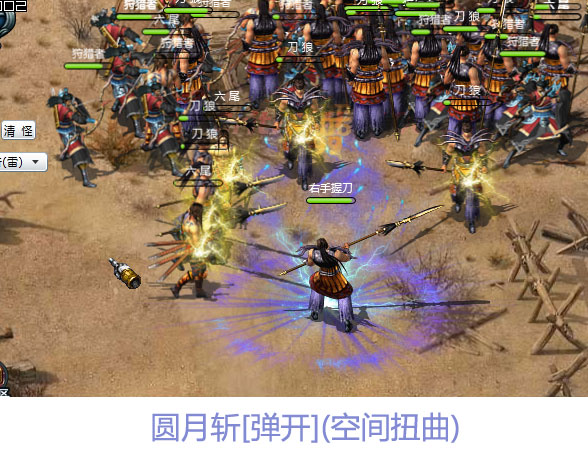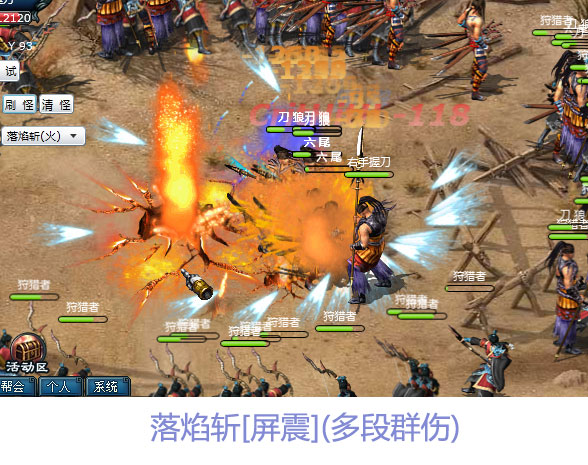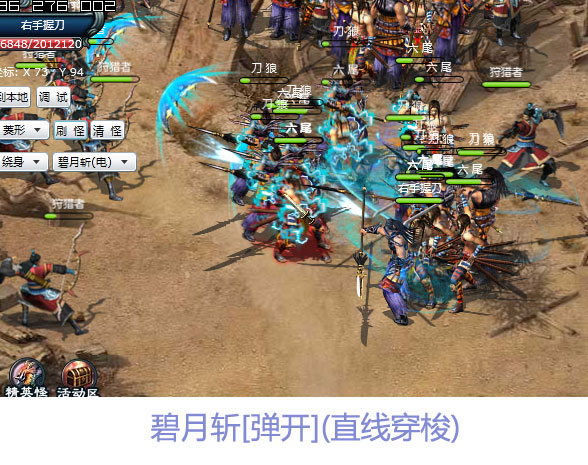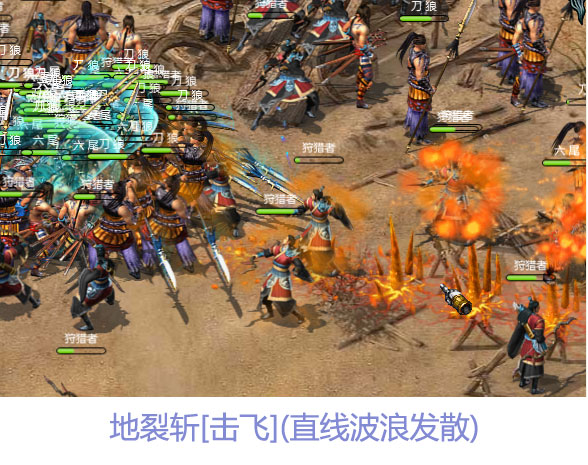using System.Collections.Generic;
using System.Windows;
using System.Reflection;

namespace Controls.Base {

#region 类

public class MagicArgs {

/// <summary>

/// 获取或设置代号

/// </summary>
public int Code { getset; }

/// <summary>

/// 获取或设置出现坐标

/// </summary>
public Point Coordinate { getset; }

/// <summary>

/// 获取或设置目标

/// </summary>
public Point Target { getset; }

/// <summary>

/// 获取或设置Z层次

/// </summary>
public int Z { getset; }

/// <summary>

/// 获取或设置等级

/// </summary>
public int Level { getset; }

/// <summary>

/// 获取或设置最小伤害

/// </summary>
public int DamageMin { getset; }

/// <summary>

/// 获取或设置最大伤害

/// </summary>
public int DamageMax { getset; }

/// <summary>

/// 获取或设置范围半径

/// </summary>
public int Radius { getset; }

/// <summary>

/// 获取或设置魔法类型

/// </summary>
public MagicTypes MagicType { getset; }

/// <summary>

/// 获取或设置装饰特效

/// </summary>
public SpecialEffects SpecialEffect { getset; }

/// <summary>

/// 获取或设置附加效果

/// </summary>

/// <summary>

/// 获取或设置数量

/// </summary>
public int Number { getset; }
}

#endregion

#region 枚举

/// <summary>

/// 魔法类型

/// </summary>
public enum MagicTypes {

/// <summary>

/// 圆形

/// </summary>
CircleMagic = 0,

/// <summary>

/// 扇形

/// </summary>
SectorMagic = 1,

/// <summary>

/// 十字

/// </summary>
CrossMagic = 2,

/// <summary>

/// 圆发散波浪

/// </summary>
CircleWaveMagic = 3,

/// <summary>

/// 圆多段

/// </summary>
MultipleHitCircleMagic = 4,

/// <summary>

/// 矩形穿梭

/// </summary>
LinearShuttleMagic = 5,

/// <summary>

/// 矩形波浪

/// </summary>
LinearWaveMagic = 6,
}

/// <summary>

/// 特殊效果

/// </summary>
public enum SpecialEffects {

/// <summary>

/// 无

/// </summary>
None = -1,

/// <summary>

/// 麻痹

/// </summary>
Mine = 7,

/// <summary>

/// 风切

/// </summary>
Wind = 8,

/// <summary>

/// 灼烧

/// </summary>
Fire = 9,

/// <summary>

/// 冰冻

/// </summary>
Ice = 10,

/// <summary>

/// 爆裂

/// </summary>
Explosion = 12,

/// <summary>

/// 爆裂1

/// </summary>
Explosion1 = 20,
}

/// <summary>

/// 附加效果

/// </summary>

/// <summary>

/// 击伤

/// </summary>
Injure = 0,

/// <summary>

/// 弹开

/// </summary>
Bounce = 1,

/// <summary>

/// 击飞

/// </summary>
BeKnock = 2,
}

#endregion

/// <summary>

/// 魔法类

/// </summary>
public abstract class MagicBase {

#region 属性

/// <summary>

/// 获取或设置参数

/// </summary>
public MagicArgs Args { getset; }

/// <summary>

/// 获取或设置目标精灵集合

/// </summary>
public List<Sprite> Targets { getset; }

#endregion

#region 构造

public MagicBase() {
Targets
= new List<Sprite>();
}

#endregion

#region 方法

/// <summary>

/// 触发/运行

/// </summary>

/// <param name="caster">施法者</param>

/// <param name="scene">所在场景</param>

/// <param name="args">魔法参数</param>
public abstract void Run(Sprite caster, Scene scene, MagicArgs args);

#endregion

}
}

//开始技能/魔法攻击
void sprite_DoCasting(object sender, DoCastingEventArgs e) {
Sprite caster
= sender as Sprite;

//通过反射来加载并实例化各类型魔法
MagicBase magic
= assembly.CreateInstance(string.Format("Controls.Magic.{0}",e.MagicArgs.MagicType.ToString())) as MagicBase;
magic.Run(caster, scene, e.MagicArgs);
}

到此，整个Controls控件项目库的结构已脉络清晰，关系图如下：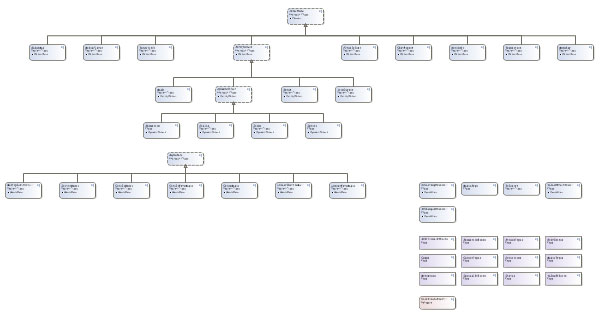1、  通过独立存储来保存和配置。

2、  也是本节源码中我所重构的方案，即将每个精灵的资源独立存放在新建的Silverlight应用程序中，这样编译后将产生对应包含该精灵资源的XAP文件，需要时通过动态下载XAP再读取其中图片的方法呈现精灵。过程中需要将资源缓存到内存中以保存这些图片的数据流，相对之前的那些方案，该方案在性能和内存方面都可以达到一个折中且偏上的效果，不仅维护方便，且对于资源来说也起到一定的保密作用：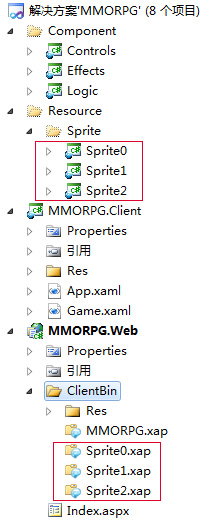1、改进及优化动态障碍物系统（包括其中的障碍物碰撞检测、以及精灵AI）。

2、重新封装游戏对象的3个坐标属性以满足各种情况需求:窗口坐标系精确值，游戏坐标系精确值，游戏坐标系整值。

3、编写更多的游戏开发辅助工具以提升效率，比如游戏资源管理器等。

4PNG图片格式在未来软件应用中显得尤为重要，包括8位和24位，甚至它的动画格式MNG，因为无论是2D还是3D；无论是SilverlightFlash还是HTML5；无论是在网页上还是客户端亦或是WP7PNG格式将在未来的2D图像呈现方面长期处于统治地位。

5、更多技巧需要相互的取长补短以习得，做游戏就得从玩游戏开始一点没错。强烈推荐开发者去参考目前已运营的商业游戏，利用浏览器缓存查看它们的资源布局、配置存储、压缩加密以及动画的处理手法等等，高手均诞生于普通人之中，只是看得多了，做的多了就成了师傅，尔耳。

[一期]结束语：一款成功的商业游戏必须具备天时、地利、人和。优秀的美工团队意味着游戏成功了一半，这绝非危言耸听；而像我们这类做程序的则常挂嘴边最多的词不外呼“性能”二字，回归原点，性能的关键取决于细节。完美的画面加上优秀的游戏架构和引人入胜的体验将缔造成功参数中的“人和”，假以时日在那即将载入史册的场景，旷世之巨作莅临终将显得毫无悬念。

Silverlight网游开发真诚期待您的加入，行动吧！那就从今天开始。

()

posted on 2010-12-22 22:39  深蓝色右手  阅读(6911)  评论(29编辑  收藏